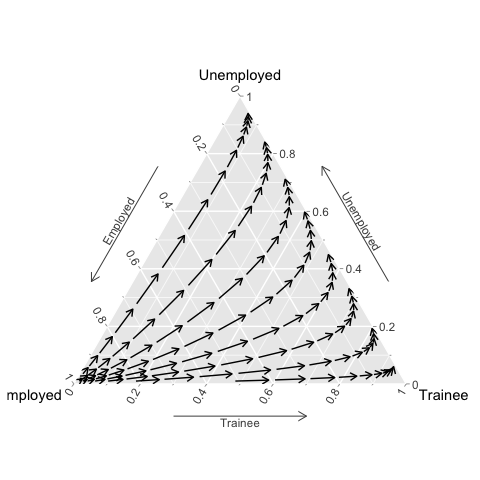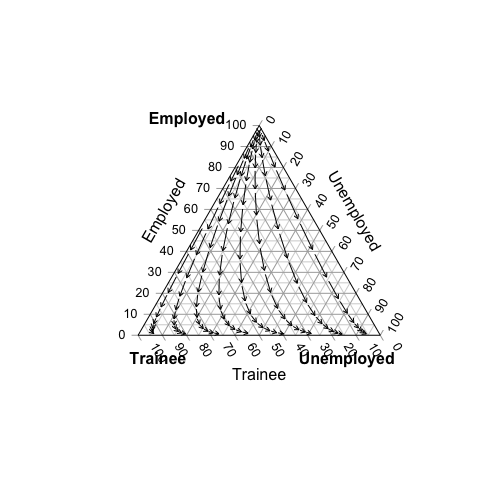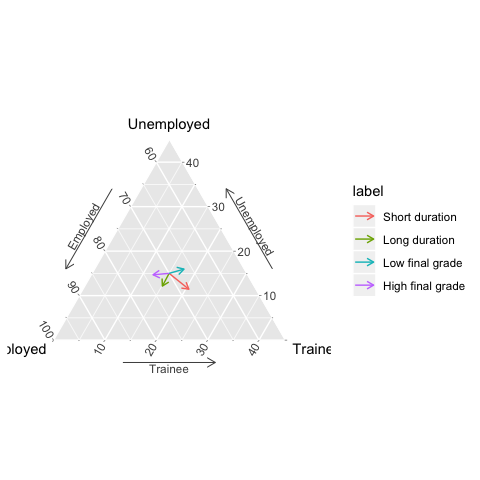# An overview of package plot3logit

## Introduction

The package permits the covariate effects of trinomial regression models to be represented graphically by means of a ternary plot. The aim of the plots is helping the interpretation of regression coefficients in terms of the effects that a change in regressors’ values has on the probability distribution of the dependent variable. Such changes may involve either a single regressor, or a group of them (composite changes), and the package permits both cases to be handled in a user-friendly way. Theoretical and methodological details are illustrated and discussed in Santi, Dickson and Espa (2019).

The package can read the results of both categorical and ordinal trinomial logit regression fitted by various functions (see the next section) and creates a field3logit object which may be represented by means of functions gg3logit and stat_3logit.

The plot3logit package inherits graphical classes and methods from the package ggtern (Hamilton 2018) which, in turn, is based on the package ggplot2 (Wickham 2016).

Graphical representation based on standard graphics is made available through the package Ternary (Smith 2017) by functions plot3logit and TernaryField, and by the plot method of field3logit objects.

See the help of field3logit for representing composite effects and multifield3logit for drawing multiple fields.

## Compatibility

Function field3logit of package plot3logit can read trinomial regression estimates from the output of the following functions:

• multinom of package nnet (logit regression);
• polr of package MASS (ordinal logit regression);
• mlogit of package mlogit (logit regression).

Moreover, explicit matrix of regression coefficients can be passed to field3logit. See the help of the package (type ? 'plot3logit-package') for furhter details.

## A brief example

Fit a trilogit model by means of package nnet where the student’s employment situation is analysed with respect to all variables in the dataset cross_1year:

library(plot3logit)
data(cross_1year)
library(nnet)
mod0 <- multinom(employment_sit ~ ., data = cross_1year)
#> # weights:  42 (26 variable)
#> initial  value 3605.645531
#> iter  10 value 2167.042903
#> iter  20 value 2136.782685
#> iter  30 value 2134.363158
#> final  value 2134.352162
#> converged

The gender effect is analysed by means of a ternary plot which is generated in two steps, however, package plot3logit should be loaded:

library(plot3logit)

Firstly, the vector field is computed:

field0 <- field3logit(mod0, 'genderFemale')

Secondly, the field is represented on a ternary plot, using either gg-graphics:

gg3logit(field0) + stat_3logit()or standard graphics:

plot(field0)## How to specify the covariate changes

Ternary plots represent the effect of a change in covariate values on the probability distribution of the dependent variable. The function field3logit permits such change to be specified in two different ways, which are illustrated below.

As an example, the following subsections refer to this dataset:

data(cross_1year)
str(cross_1year)
#> 'data.frame':    3282 obs. of  7 variables:
#>  $employment_sit: Factor w/ 3 levels "Employed","Unemployed",..: 1 1 1 3 1 1 1 1 1 2 ... #>$ gender        : Factor w/ 2 levels "Male","Female": 2 1 2 2 2 1 2 1 1 2 ...
#>  $finalgrade : Factor w/ 3 levels "Average","Low",..: 3 3 1 1 2 3 1 1 2 3 ... #>$ duration      : Factor w/ 3 levels "Average","Short",..: 3 3 3 3 3 3 1 3 3 3 ...
#>  $social_class : Factor w/ 5 levels "Working class",..: 1 2 4 4 3 3 1 4 1 2 ... #>$ irregularity  : Factor w/ 3 levels "Average","Low",..: 1 1 3 3 3 3 1 3 3 3 ...
#>  \$ hsscore       : num  100 95 82 64 69 ...
#>   employment_sit gender finalgrade duration         social_class
#> 1       Employed Female       High     Long        Working class
#> 2       Employed   Male       High     Long White-collar workers
#> 3       Employed Female    Average     Long   Upper middle class
#> 4        Trainee Female    Average     Long   Upper middle class
#> 5       Employed Female        Low     Long   Lower middle class
#> 6       Employed   Male       High     Long   Lower middle class
#>   irregularity   hsscore
#> 1      Average 100.00000
#> 2      Average  95.00000
#> 3         High  82.00000
#> 4         High  64.00000
#> 5         High  69.00000
#> 6         High  86.66667

and this trinomial logistic regression model:

mod0 <- nnet::multinom(employment_sit ~ finalgrade + irregularity + hsscore, cross_1year)
#> # weights:  21 (12 variable)
#> initial  value 3605.645531
#> iter  10 value 2187.709284
#> iter  20 value 2157.087955
#> final  value 2157.087854
#> converged
mod0
#> Call:
#> nnet::multinom(formula = employment_sit ~ finalgrade + irregularity +
#>     hsscore, data = cross_1year)
#>
#> Coefficients:
#> Unemployed  -0.4481761    0.05551765    -0.07810893     -0.01874354
#> Trainee     -1.3751140    0.14456683    -0.26849829      0.05764144
#>            irregularityHigh      hsscore
#> Unemployed       0.15691595 -0.016619227
#> Trainee         -0.03477569 -0.009964381
#>
#> Residual Deviance: 4314.176
#> AIC: 4338.176

### Explicit specification of $$\Delta x$$

This method for specifying the change in the covariate values requires the vector $$\Delta x$$ to be explicitly defined, thus it may be suitable when $$\Delta x$$ results from some calculations. On the other hand, it is less user-friendly than implicit syntax, as it depends on the order of regressors in the design matrix.

#### First example

If the effect of a high final grade has to be assessed, the vector of changes $$\Delta x$$ can be set according to the position of the dummy variable finalgradeHigh in the matrix of coefficients of the model mod0:

coef(mod0)
#> Unemployed  -0.4481761    0.05551765    -0.07810893     -0.01874354
#> Trainee     -1.3751140    0.14456683    -0.26849829      0.05764144
#>            irregularityHigh      hsscore
#> Unemployed       0.15691595 -0.016619227
#> Trainee         -0.03477569 -0.009964381

in this case, we have that $\Delta x=[0, 0, 1, 0, 0, 0]'$ since finalgradeHigh is the fourth coefficient (including the intercept) of the matrix of coefficients.

It follows that the function field3logit can be invoked as it follows:

field0 <- field3logit(mod0, c(0, 0, 1, 0, 0, 0))
field0
#>  Object of class "field3logit"
#> -------------------------------
#> Label                    : <empty>
#> Possible outcomes        : Employed; Unemployed; Trainee
#> Effect                   : 0 0 1 0 0 0
#> Model has been read from : nnet::multinom
#> Number of curves         : 8
#> Number of arrows         : 182
#> Covariance matrix        : available

#### Second example

It is also possible to set $$\Delta x$$ so as to consider changes involving more than one regressor, as well as fractional changes. In such cases, $$\Delta x$$ will consist in a vector where there are several non-zero elements which may take any positive or negative value.

Assume, for example, that we want to study the effect of a decrease by 10 in the high school final score, associated to an high final grade. In such a case, we have that: $\Delta x =[0, 0, 1, 0, 0, -10]'\,,$ thus:

field0 <- field3logit(mod0, c(0, 0, 1, 0, 0, -10))
field0
#>  Object of class "field3logit"
#> -------------------------------
#> Label                    : <empty>
#> Possible outcomes        : Employed; Unemployed; Trainee
#> Effect                   : 0 0 1 0 0 -10
#> Model has been read from : nnet::multinom
#> Number of curves         : 8
#> Number of arrows         : 166
#> Covariance matrix        : available

### Implicit specification of $$\Delta x$$

Unlike the explicit method, this syntax allows the user to initialise the vector $$\Delta x$$ by writing the name of the covariate which should vary: the function field3logit will build $$\Delta x$$ up associated to a unitary change in the specified covariate.

If more complex changes in covariate values have to be considered, implicit syntax allows the user to express them in terms of R expressions involving the covariates.

#### First example

If the effect of a high final grade has to be assessed, the implicit syntax which allow to assess the effect of a unitary change of finalgradeHigh is the following:

field0 <- field3logit(mod0, 'finalgradeHigh')
field0
#>  Object of class "field3logit"
#> -------------------------------
#> Label                    : <empty>
#> Possible outcomes        : Employed; Unemployed; Trainee
#> Explicit effect          : 0 0 1 0 0 0
#> Model has been read from : nnet::multinom
#> Number of curves         : 8
#> Number of arrows         : 182
#> Covariance matrix        : available

Note that the console output produced by printing field0 shows both the implicit effect (line Effect) and the associated vector $$\Delta x$$ (line Explicit effect).

#### Second example

If we want to study the effect of a decrease by 10 in the high school final score, associated to an high final grade, the implicit syntax is:

field0 <- field3logit(mod0, 'finalgradeHigh - 10 * hsscore')
field0
#>  Object of class "field3logit"
#> -------------------------------
#> Label                    : <empty>
#> Possible outcomes        : Employed; Unemployed; Trainee
#> Effect                   : finalgradeHigh - 10 * hsscore
#> Explicit effect          : 0 0 1 0 0 -10
#> Model has been read from : nnet::multinom
#> Number of curves         : 8
#> Number of arrows         : 166
#> Covariance matrix        : available

Compare the line Explicit effect of this output to the line Effect of the same example in the previous section: as expected, they are the same.

## Comparing multiple effects

When effects of multiple changes have to be compared at a time, multiple fields should be computed and represented on the same plot. This task can be easily done by creating a multifield3logit object and directly representing it.

Since objects multifield3logit result by putting together two or more field3logit objects, the package plot3logit allows the user to create a multifield3logit object by adding up two or more filed3logit or multifield3logit objects using standard sum operator +.

Here it is an example. The following command fit a trilogit model where all available variables are used as regressors. Then four fields3logit objects are computed for assessing the effects of a some combined changes in the duration of studies and in students’ final degree score.

Note that each field is computed just with respect to a single probability distribution (refpoint) of the dependent variable, and only one arrow is computed. The reason of this is that we have to represent four fields on the same plot, thus olny a small number of arrows can be drawn in order to preserve the clarity of the graph.

data(cross_1year)
mod0 <- nnet::multinom(employment_sit ~ ., data = cross_1year)

refpoint <- list(c(0.7, 0.15, 0.15))

field_Sdur <- field3logit(mod0, 'durationShort', label = 'Short duration', p0 = refpoint, narrows = 1)
field_Ldur <- field3logit(mod0, 'durationLong', label = 'Long duration', p0 = refpoint, narrows = 1)
field_Hfgr <- field3logit(mod0, 'finalgradeHigh', label = 'High final grade', p0 = refpoint, narrows = 1)
field_Lfgr <- field3logit(mod0, 'finalgradeLow', label = 'Low final grade', p0 = refpoint, narrows = 1)

Now the multifield3logit object can be created by adding all the field3logit objects up together:

mfields <- field_Sdur + field_Ldur + field_Lfgr + field_Hfgr
mfields
#>  Object of class "multifield3logit"
#> ------------------------------------
#> Number of fields         : 4
#> Labels
#>   1. Short duration    (dX: durationShort)
#>   2. Long duration     (dX: durationLong)
#>   4. High final grade  (dX: finalgradeHigh)
and the multifield3logit object mfield can be represented in a graph:
gg3logit(mfields) +
theme_zoom_L(0.45)